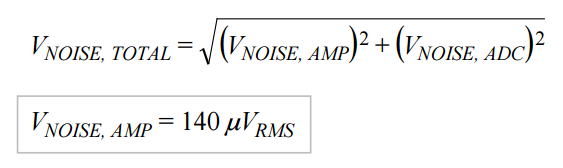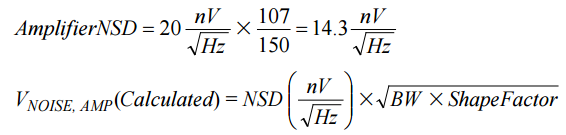登録が必要です

## myAnalogのパスワードを作成し、ログインしてこの記事にアクセスしてください。

1. 8-16 文字の英数字
2. 以下の中から 3 種類の文字を使用してください:
• 小文字
• 大文字
• 数字 (0-9)
• 以下の中から一つ以上の記号:
@ # \$ % ^ & * - _ + = [ ] { } | \ : ' , ? / ` ~ " ( ) ; .

# Understanding How Amplifier Noise Contributes to Total Noise in ADC Signal Chains

### Introduction

Analog-to-digital converters (ADCs) provide optimal performance when the analog inputs are driven to their rated full-scale input voltage, but in many applications, the maximum available signal differs from the specified voltage and may need to be adjusted. A useful device for handling this requirement is a variable-gain amplifier (VGA). Understanding how the VGA affects the ADC’s performance will help in optimizing performance of the entire signal chain.

This article analyzes noise in a circuit that uses the AD9268 dual 16-bit, 125-/105-/80-MSPS, pipelined ADC, and the AD8375 ultralow distortion IF VGA. The signal chain includes a VGA—used at a gain setting of +6 dB, a 5th-order Butterworth low-pass filter with a –3-dB roll-off at 100 MHz, and the ADC. Noise calculations will be shown for the amplifier and filter, as they dictate the dynamic performance of the ADC over the band of interest.

### Problem

Many real-world applications using high-speed ADCs need some sort of driver, amplifier, or gain block that scales the input signal to the full-scale analog input range1 to ensure optimum signal-to-noise ratio (SNR) and spurious-free dynamic range (SFDR). In addition, a differential amplifier could also convert a single-ended signal to a differential signal to drive the ADC. Being active, these components contribute noise at the ADC front end. The integration of this noise over the operational bandwidth will degrade the conversion performance.

The choice of an appropriate ADC for an application depends on many factors, including:

• Input frequency/bandwidth
• Desired resolution/SNR
• Desired SFDR

Some applications require both high dynamic range and high resolution. The AD9268 is very good choice for these applications, delivering 78.2-dBFS (dB relative to full-scale) SNR and 88-dBc SFDR at a 70-MHz intermediate frequency.

At the system level, the ADC front-end could use an amplifier, a transformer, or a balun, but implementations using an amplifier are most common. An amplifier could be used for one or more of the following reasons:

• Provide gain to the input signal to increase the ADC resolution.
• Buffer or transform the impedance between the input source and the ADC.
• Convert a single-ended input signal to a differential output signal.

The AD8375 VGA, which maintains high linearity and uniform noise performance over its various gain settings, can be used to convert single-ended signals to differential. These characteristics make it a good candidate for driving the ADC at higher intermediate frequencies. Unfortunately, the presence of an active device—the amplifier—in the signal chain can limit the ADC’s performance.

### Example

Figure 1 shows the circuit topology used in performing the noise calculations. The AD8375 has a high-impedance differential output (16 kΩ||0.8 pF). A 5th-order low-pass antialiasing filter (AAF) with 100-MHz bandwidth and 150-Ω input and output impedances interfaces the amplifier to the ADC. The frequency response of the circuit shown in Figure 1 can be seen in Figure 2.

### Performance

System designers may not expect the amplifier driving the ADC inputs to degrade the overall dynamic performance of the system, but the fact that a driver and ADC combo was chosen for one application does not mean that it will provide the same great performance in another application. The technique described here allows a systems engineer to estimate the expected performance before choosing an amplifier.

Figure 3 shows two different setups: Figure 3(a), which uses passive coupling to the converter, is available as the default option on the customer evaluation board. The passive front-end network uses a transformer or balun to convert the single-ended signal to differential, along with a passive low-pass filter that rolls off at about 200 MHz. Figure 3(b) shows the optional amplifier path. A comparison of the noise contributed by these two setups follows. A single-tone fast Fourier transform (FFT) at a low intermediate frequency (10 MHz) is used to calculate the noise added by the amplifier.

Two techniques are typically used for analyzing the noise, but each can be cumbersome. Noise spectral density (NSD) defines the noise power per unit bandwidth. It is represented in mean-square dBm/Hz or dBFS/Hz for ADCs and rms nV/√Hz for amplifiers. This incompatibility in units provides an obstacle to calculating system noise when an amplifier is driving an ADC.

Noise figure (NF) is the log ratio of input SNR to the output SNR expressed in decibels. This specification, commonly used by RF engineers, makes sense in a purely RF world, but attempting to use NF calculations in a signal chain with an ADC can lead to misleading results.2

An alternate, but more effective, technique is to “de-normalize” the noise density, representing it as an rms noise voltage rather than a mean-square voltage. This method, as described here, is straightforward and allows a clear analysis of the system noise.

Figure 4 and Figure 5 show the low-frequency single-tone FFTs of the two front ends. Note that the passive front end has 77.7-dBFS SNR, while the active front end has 72.5-dBFS SNR, 5.2 dBFS lower than the ADC’s expected performance.

### Analysis

The only difference between the setups shown in Figure 3a and 3b is the addition of the amplifier in the signal chain, so it is safe to assume that the performance degradation is caused by the amplifier’s noise. The following calculations help to understand the additional noise introduced by the amplifier.

First, use the converter’s full-scale differential input voltage, as specified in the data sheet. Convert the peak-to-peak voltage to rms by dividing by 2√2 to get 0.707 V rms.(1)

The contribution of the converter’s noise based on the ADC’s typical SNR at 10 MHz is(2)(3)

Using VNOISE, ADC = 92.2 μV rms and system SNR with the amplifier front end = 72.5 dBFS, the system noise, using Equation 3, is 168 μV rms.(4)(5)

The system noise obtained from Equation 4 is the combined noise of the ADC and the VGA. The amplifier noise can be calculated from Equation 5 to be 140 μV rms. This calculation shows that the amplifier noise is at least 50% greater than the ADC noise, making it the limiting factor in determining the system’s ac performance.

Note that we must determine whether the value of VNOISE, AMP calculated above matches the amplifier’s data sheet. The specified noise spectral density is about 20 nV/√Hz with a 150-Ω differential output impedance.

Although the data sheet specifies that the VGA’s noise is fairly constant with gain, this noise will change with the load, so the noise spectral density should be scaled to the total impedance driven by the amplifier outputs. Because the differential output impedance of the amplifier is large (16 kΩ||0.8 pF), the impedance seen by the amplifier (see Figure 1) can be calculated as

[10 Ω + (300 Ω||150 Ω||3.5 kΩ)] = 107 Ω.

Using this number, the derated noise spectral density for the AD8375 in this application can be found from Equation 6:(6)

Note that when calculating system noise using a real filter, the noise bandwidth takes on a different shape than that of an ideal filter. This deviation in frequency response is characterized by the term, shape factor, and accounts for the noise in the roll-off region. The shape factor, which depends on the order of the filter, is the ratio of the noise bandwidth to the –3-dB bandwidth.3 The more poles in the filter, the closer the shape factor is to unity. This relationship can be seen in Table 1.

Table 1. Relationship Between System Order and Shape Factor

 System Order Shape Factor 1 1.57 2 1.11 3 1.05 4 1.03 5 1.02

In the example of Figure 1, the shape factor is 1.02. Using Equation 6, the noise injected by the amplifier will be;

This estimated value of noise injected into the system by the VGA matches very well with the measured value using Equation 5, proving that the performance of the signal chain comprising the AD8375 and AD9268 is dominated by the amplifier.

### Conclusion

In many cases, an amplifier—VGA or gain block—is required to drive a full-scale signal to an ADC in the system signal chain. The systems designer must be aware of the degradation in optimal performance of the ADC caused by the choice of the amplifier. Before designing with the chosen amplifier and ADC, the designer can use the method shown here to calculate the noise contribution of the amplifier to estimate the expected dynamic performance, as characterized by the SNR, for the intended system implementation.

I invite you to comment on noise in ADC signal chains in the Analog Dialogue Community on EngineerZone.

### 参考資料

3Reeder, Rob and Jim Caserta, “Ask The Application Engineer 36, Wideband A/D Converter Front-End Design Considerations II: Amplifier-or Transformer Drive for the ADC?” Analog Dialogue 41-02, 2007.